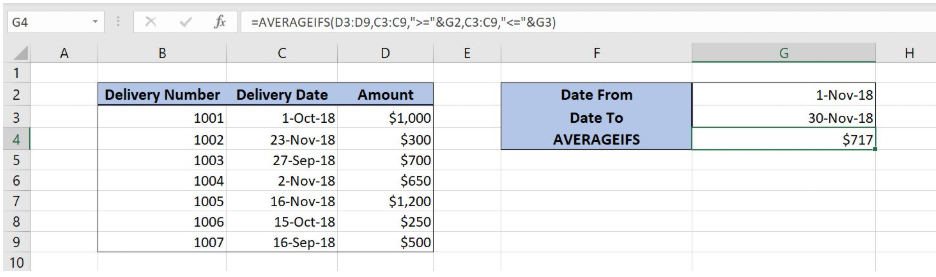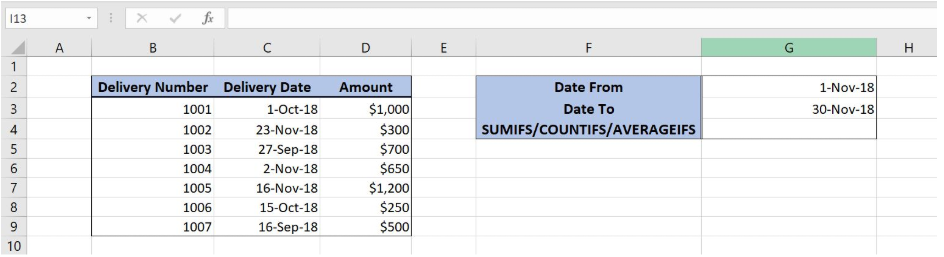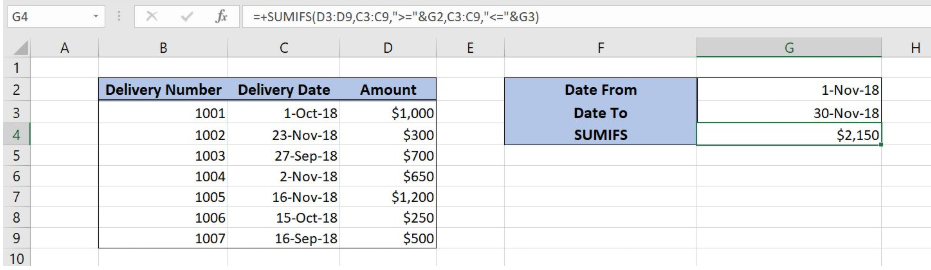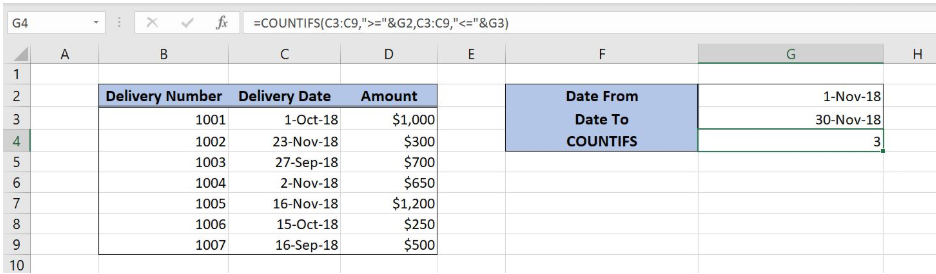Get instant live expert help with Excel or Google Sheets“My Excelchat expert helped me in less than 20 minutes, saving me what would have been 5 hours of work!”

#### Post your problem and you’ll get Expert help in seconds.

Your message must be at least 40 characters
Our professional Expert are available now. Your privacy is guaranteed.

# Comparing SUMIF vs COUNTIF vs AVERAGEIFS to Deal with Multiple Criteria

Excel can calculate a sum, average or count of values based on specific criteria by using the SUMIFS, COUNTIFS and AVERAGEIFS functions. This step by step tutorial will assist all levels of Excel users in comparing these functions to deal with multiple criteria.Figure 1. Final result

## Syntax of the SUMIFS formula

`=SUMIFS(sum_range, criteria_range1, criteria1, criteria_range2, criteria2)`

The parameters of the SUMIFS function are:

• sum_range – a range with values which we want to sum
• criteria_range1 – a range where we want to set our first condition
• criteria1 – the first condition for summing the values
• criteria_range2 – a range where we want to set our second condition
• criteria2 – the second condition for summing the values

## Setting up Our Data for the Functions

Our table consists of 3 columns: “Delivery Number” (column B), “Delivery Date” (column C) and “Amount” (column C). In cells G2 and G3, we specify a date range, while in cell G4 we want to get a result of the function.Figure 2. Data that we will use in the examples

## Sum Amount Between Two Value Ranges Using the SUMIFS Function

In our example, we want to sum all amounts from column D that are between 1-Nov-18 and 30-Nov-18.

### Formula:

`=SUMIFS(D3:D9, C3:C9, ">="&G2, C3:C9, "<="&G3)`

The sum_range is D3:D9. Criteria1 is “>=”&G2. As our first criteria is the date greater than or equal to G2 (1-Nov-18). Criteria2 is “<=”&G3

To apply the SUMIFS function, we need to follow these steps:

• Select cell G4 and click on it
• Insert the formula: `=SUMIFS(D3:D9, C3:C9, ">="&G2, C3:C9, "<="&G3)`
• Press enterFigure 3. Using the SUMIFS function to sum values between two dates

In this example, we get all amounts which have the corresponding date between 1-Nov-18 and 30-Nov-18. As you can see, rows 4 (23-Nov-18), 6 (2-Nov-18) and 7 (16-Nov-18) meet both conditions, so corresponding amounts are summed (\$300, \$650, \$1,200). Finally, the sum in the cell G4 is \$2,150.

## Syntax of the COUNTIFS formula

`=COUNTIFS(criteria_range1, criteria1, criteria_range2, criteria2)`

The parameters of the COUNTIFS function are:

• criteria_range1 – a range where we want to set our first condition
• criteria1 – the first condition for summing the values
• criteria_range2 – a range where we want to set our second condition
• criteria2 – the second condition for summing the values

## Count Items Between Two Value Ranges Using the COUNTIFS Function

In our example, we want to count all items that are between 1-Nov-18 and 30-Nov-18.

### Formula:

`=COUNTIFS(C3:C9,">="&G2,C3:C9,"<="&G3)`

Both Criteria_range1 and Criteria_range2 are the same – C3:C9 (Delivery Date). Criteria1 is “>=”&G2. As our first criteria is the date greater than or equal to G2 (1-Nov-18). Criteria2 is “<=”&G3

To apply the COUNTIFS function, we need to follow these steps:

• Select cell G4 and click on it
• Insert the formula: `=COUNTIFS(C3:C9,">="&G2,C3:C9,"<="&G3)`
• Press enterFigure 4. Using the COUNTIFS function to count items between two dates

In this example, the function will calculate all the corresponding date between 1-Nov-18 and 30-Nov-18. As you can see, rows 4 (23-Nov-18), 6 (2-Nov-18) and 7 (16-Nov-18) meet both conditions, so the count in the cell G4 is 3.

## Syntax of the AVERAGEIFS formula

`=AVERAGEIFS(average_range, criteria_range1, criteria1, criteria_range2, criteria2)`

The parameters of the AVERAGEIFS function are:

• average_range – a range with values for which we want to calculate the average
• criteria_range1 – a range where we want to set our first condition
• criteria1 – the first condition for calculating the average of the values
• criteria_range2 – a range where we want to set our second condition
• criteria2 – the second condition for calculating the average of the values

## Calculate the Average Between Two Value Ranges Using the AVERAGEIFS Function

In our example, we want to calculate the average of all the amounts from column D that are between 1-Nov-18 and 30-Nov-18.

### Formula:

`=AVERAGEIFS(D3:D9, C3:C9, ">="&G2, C3:C9, "<="&G3)`

The average_range is D3:D9. Criteria1 is “>=”&G2. As our first criteria is the date greater than or equal to G2 (1-Nov-18). Criteria2 is “<=”&G3

To apply the AVERAGEIFS function, we need to follow these steps:

• Select cell G4 and click on it
• Insert the formula: `=AVERAGEIFS(D3:D9, C3:C9, ">="&G2, C3:C9, "<="&G3)`
• Press enterFigure 5. Using the AVERAGEIFS function to calculate the average of the amounts between two dates

In this example, all the amounts that have the corresponding date between 1-Nov-18 and 30-Nov-18 are taken into the calculation of the average. As you can see, rows 4 (23-Nov-18), 6 (2-Nov-18) and 7 (16-Nov-18) meet both conditions, so correspondings amounts are summed (\$300, \$650, \$1,200) and divided by 3. Finally, the average in the cell G4 is \$717.

Most of the time, the problem you will need to solve will be more complex than a simple application of a formula or function. If you want to save hours of research and frustration, try our live Excelchat service! Our Excel Experts are available 24/7 to answer any Excel question you may have. We guarantee a connection within 30 seconds and a customized solution within 20 minutes.

### Did this post not answer your question? Get a solution from connecting with the expert.Another blog reader asked this question today on Excelchat:
Solution examplesI am trying to utilize a complicated sumif or sumifs formula that can pull a total amount from a date and a drop down box from another tab.
Solved by E. B. in 15 minsI have a spreadsheet that totals invoices. A bunch of cells at the bottom of the spreadsheet calculates totals based on which department the invoice is charged to. I'd like to add a function where it also considers the date (so if it's an invoice in January, it goes to a January total, February, etc.). But I can't figure out how to write it properly...
Solved by K. J. in 27 minsIf I want to write a formula that states" "If this name shows up K24, and this word shows up in J24 pull this number from M24" I can creat that formula - however I need to drag this down so it pulls from row 24 - 60 - is there way to do this?
Solved by F. D. in 40 minsi need a formula to find values in column f in worksheet 1 and count them.
Solved by B. A. in 20 minshow can i turn my sumifs into a countifs because my sumifs works great and i also want to count the thing im summing
Solved by O. C. in 40 mins## Subscribe to Excelchat.coAnother blog reader asked this question today on Excelchat: